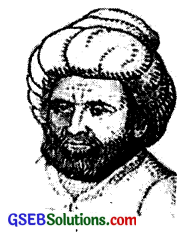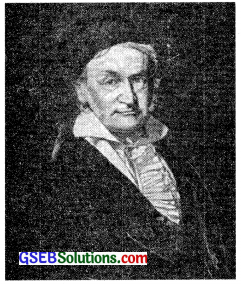# GSEB Class 10 Maths Notes Chapter 1 Real Numbers

This GSEB Class 10 Maths Notes Chapter 1 Real Numbers covers all the important topics and concepts as mentioned in the chapter.

## Real Numbers Class 10 GSEB Notes

Euclid’s division algorithm, as the name suggests, has to do with divisibility of integers. Euclid says any positive integer a can be divided by another positive integer b in such a way that it leaves a remainder r that is smaller than b. Many of us probably recognise that as the usual long division process. Although this result is quite easy to state and understand, it has many applications related to the divisibility properties of integers.

The Fundamental Theorem of Arithmetic, has to do with multiplication of positive integers. Every composite number can be expressed as a product of primes in a unique way-this important fact is the fundamental theorem of arithmetic.

Fundamental Theorem of Arithmetic has two main applications. Firstly, we use it to prove the irrationality of many of the numbers such as $$\sqrt{2}$$ , $$\sqrt{3}$$ , $$\sqrt{5}$$ etc. Secondly, we apply this theorem to explore when exactly the decimal expansion of a rational number, say $$\frac{p}{q}$$(9 ≠ 0), is terminating and when it is non-terminating repeating. We do it by the prime factorisation of q will completely reveal the nature of the decimal expansion of $$\frac{p}{q}$$.

Theorem 1.1.
Euclid’s Division Lemma
Given positive integers a and b, there exist unique whole numbers q and r satisfying
a = bq + r, 0 ≤ r < b.

In simple words,
Dividend = (Divisor) (Quotient) + Remainder

An algorithm is a series of well defined steps which gives a procedure for solving a type of problem.
The word algorithm comes from the name of the 9th century Persian mathematician al-Khwarizmi. In fact, even the word ‘algebra’ is derived from a book, he wrote, called Hisab al-jabr w’al-muqabala.
A lemma is a proven statement used for proving another statement.Muhammad ibn Musa al-Khwarizmi [A.D. 780 – 850]

Euclid’s division algorithm
To obtain the HCF of two positive integers say c and d, with c > d, follow the steps below:
Step 1.
Apply Euclid’s Lemma, to q and r, we find whole numbers, q and r such that c = dq + r ; 0 ≤ r < d.

Step 2.

• If r = 0, then d is the HCF of c and d.
• If r ≠ 0, then again apply the division Lemma to d and r.

Step 3.
Continue the process till the remainder is zero i.e., repeat the step 2 again and again untill we get remainder (r) zero. Then, the divisor at this stage will be the required HCF

Important Remarks:

• Euclid’s division algorithm is not only useful for calculating the HCF of very large numbers, but also it is one of the earliest examples of an algorithm that a computer had been programmed to carry out.
• Euclid’s division lemma and algorithm are so closely interlinked that people often call former as the division algorithm also.
• Although Euclid’s Division/Algorithm is stated for only positive integers, it can be extended for all integers except zero, i.e., b ≠ 0.
• Let c and d be two positive integers such that c = dq + r ≤ 0 < r < d then
HCF (c, d) = HCF (d, r).
• For any integer m, HCF (m, 0) = m.Theorem 1.2.
(Fundamental Theorem of Arithmetic):
Every composite number can be expressed (factorised) as a product of primes, and this factorisation is unique, apart from the order in which the prime factors occur.

An equivalent version of Theorem 1.2 was probably first recorded as Proposition 14 of Book IX in Euclid’s Elements, before it came to be known as the Fundamental Theorem of Arithmetic. However, the first correct proof was given by Carl Friedrich Gauss in his Disquisitiones Arithmeticae.Carl Friedrich Gauss (1777 – 1855)

Carl Friedrich Gauss is often referred to as the ’Prince of Mathematicians’ and is considered one of the three greatest mathematicians of all time, along with Archimedes and Newton. He had made fundamental contributions to both mathematics and science.

The Fundamental Theorem of Arithmetic says that given any composite number it can be factorised as a product of prime numbers in a ‘unique’ way, except for the order in which the primes occur. That is, given any composite number there is one and only one way to write It as a product of primes, as long as we are not particular about the order in which the primes occur.

Important Remark:

• Relationship between HCF and LCM
• For any two positive integers a and b
• HCF (a, b) × LCM (a, b) = a × b.

Theorem 1.3.
Let p be a prime number. If p divides a2, then p divides a, where a is a positive integer.
Proof:
Let the prime factorization of a be as follows: a = p1, p2…………..pn where p1, p2, ………pn are primes, not necessarily distinct.
Therefore a2 = (p1, p2,………pn) (p1, p2……….pn)

Now, we are given that p divides a2.
Therefore, from the Fundamental Theorem of Arithmetic, it follows that p is one of the prime factors of a2. However, using the uniqueness part of the Fundamental Theorem of Arithmetic, we realise that the only prime factors of a2 are
P1, P2………. Pn. So p is one P1, P2………. Pn.

Now, since a = p1, p2 …..pn, p divides a.

Theorem 1.4.
$$\sqrt{2}$$ is irrational.
Proof:
Let us assume, to the contrary, that & is rational.
So, we can find integer r and s (≠ 0) such that $$\sqrt{2}=\frac{r}{s}$$
Suppose r and s have a common factor other than 1.

Then, we divide by the common factor a to get $$\sqrt{2}=\frac{a}{b}$$, where a and b are coprime.
So, b $$\sqrt{2}$$ = a
Squaring both sides and rearranging, we get
2b2 = a2.
Therefore, 2 divides a2.
Therefore, by Theorem 1.3, it follows that 2 divides a.
So, we can write a = 2c for some integer c.

Substituting for a, we get 2b2 = 4c2, that is, b2 = 2c2.
This means that 2 divides b2, and so 2 divides b (again using Theorem 1.3 with p = 2).
Therefore, a and b have at least 2 as a common factor.

But this contradicts the fact that a and b have no common factors other than 1.
This contradiction has arisen because of our incorrect assumption that $$\sqrt{2}$$ is rational.
So, we conclude that $$\sqrt{2}$$s irrational.

Example:
Prove that $$\sqrt{3}$$ is irrational. Solution. Let us assume, to the contrary, that $$\sqrt{3}$$ is rational.
That is, we can find integers a and b (≠ 0) a
such that $$\sqrt{3}=\frac{a}{b}$$

Suppose a and b have a common factor other than 1, then we can divide by the common factor, and assume that a and b are coprime.
So, b$$\sqrt{3}$$ = a.
Squaring on both sides, and rearranging, we get 3b2 = a2.
Therefore, a2 is divisible by 3, and by Theorem 1.3, if follows that a is also divisible by 3.
So, we can write a = 3c for some integer c.

Substituting for a, we get 3b2 = 9c2, that is, b2 = 3c2.
This means that b2 is divisible by 3, and so b is also divisble by 3 (using Theorem 1.3. with P = 3).

Therefore, a and b have at least 3 as a common factor.
But this contradicts the fact that a and b are coprime.

This contradiction has arisen because of our incorrect assumption that $$\sqrt{3}$$ is rational.
So, we conclude that $$\sqrt{3}$$ is irrational.Revisiting Rational numbers and their Decimal Expansions:
Theorem 1.5.
Let x be a rational number whose decimal expansion terminates. Then x can be expressed in the form $$\frac{p}{q}$$ where p and q are coprime, and the prime factorisation of q is of the form 2n5m, where n, m are non-negative integers.

Theorem 1.6.
Let x = $$\frac{p}{q}$$ be a rational number, such that the prime factorisation of q is of the form 2n5m, where n, m are non-negative integers. Then x has a decimal expansion which terminates.

Theorem 1.7.
Let x = $$\frac{p}{q}$$ be a rational number, such that the prime factorisation of q is not of the form 2n5m, where n, m are non-negative integers. Then, x has a decimal expansion which is non-terminating repeating (recurring).

Remark: The decimal expansion of every rational number is either terminating or non-terminating repeating.